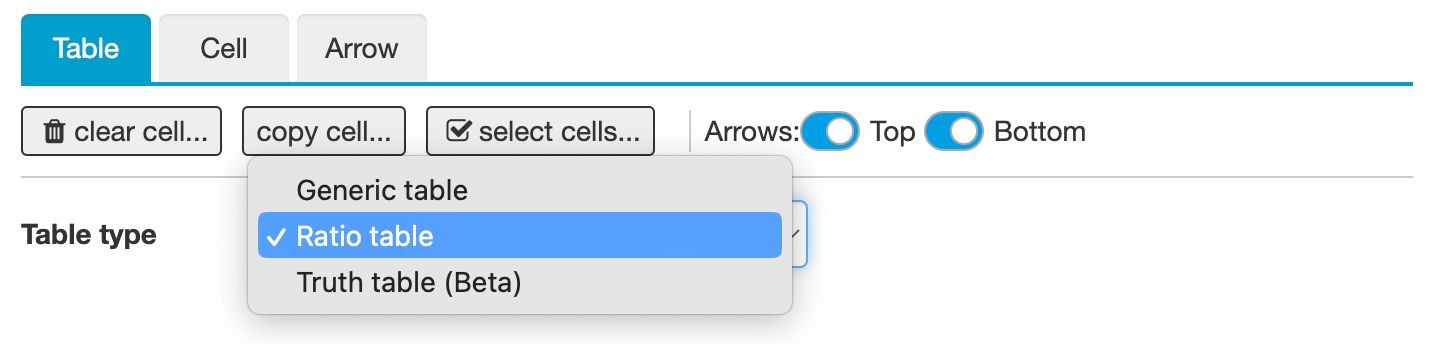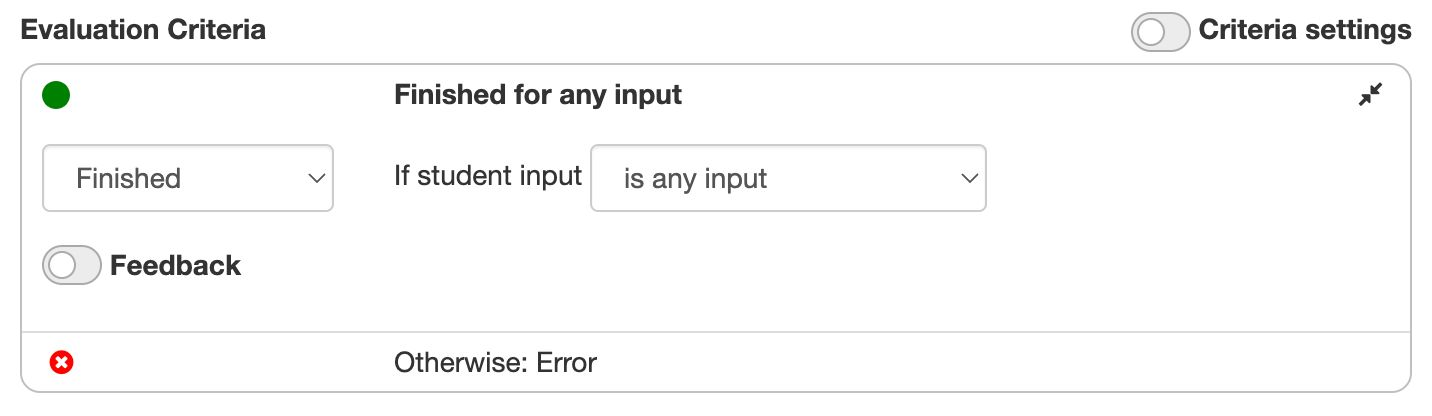# Ratio tables

## Use cases of ratio tables

Ratio tables often arise when reasoning about fractions, percentages, units, or proportional relations. There is a fixed proportionality factor between the top and bottom rows. You can also multiply columns by a constant, often visualized by arrows above and below the table.

Example: Various uses of a ratio table.

Ratio tables share all the features that other tables have. You can enable or disable arrows, preset cell values, add columns, etc. However, the table must have two rows. This means that vertical ratio tables or tables of more than two rows are unsupported.

## Enabling ratio table

Use the Table Type dropdown in the Table panel and select Ratio table. You can also enable or disable the arrows above and below the table.Figure: Enabling ratio table mode.

## Allowing any input

If you enable ratio table mode, Algebrakit will automatically check if the student input satisfies the conditions for a ratio table. This works even if you do not specify the values for each cell. You can create input cells that accept any input using evaluation criteria and choosing the option "is any input". Use the option "meets condition" to add extra conditions, such as limiting the input to natural numbers.Figure: Accepting any input on table cells.

Example: A table that accepts any input that meets the conditions of a ratio table

A problem with cells that accept any input is that Algebrakit cannot generate a solution. You can solve this by adding an extra criterium or task with specific values. Algebrakit will use these values in the solution mode while still being able to accept any input. Press the Solution button in the live example above to see how we did this.